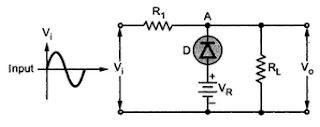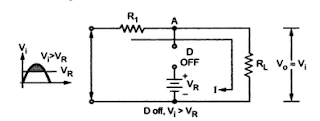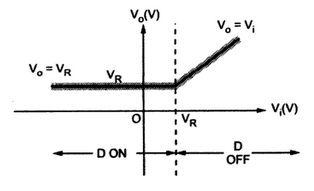### Parallel Clipper Circuits with Reference Voltage VR

If it is necessary to have reference voltage level for clipping, other than Vγ then an external battery can be used in the basic circuit.
Note : The introduction of battery in series with the diode is effective in clipping the a.c. voltage waveform above the certain reference voltage called bias voltage or reference voltage.
Reference voltage is denoted as VR. The Fig. 1 shows the basic parallel positive clipper circuit with reference voltage.Fig. 1  Positive clipper with reference voltage

1.1 Operation
The operation for positive half cycle of the input can be divided into two cases.
Case 1 : Vi is positive but less than VR
When Vi is positive but less than VR, the diode D is reverse biased and acts as open circuit. The equivalent circuit is as shown in the Fig. 2.Fig. 2  V < Vi < VR

Hence the output voltage
But selecting  R1 < <RL,
Vo = Vi                                         .......... (1)
Case 2 : Vi positive and greater than VRFig. 3   Vi  > VR

Assuming ideal diode, when Vi becomes greater than VR, the diode D becomes forward biased and acts as short circuit. This is shown in the Fig. 3. The output voltage now is same as voltage of node A which is VR, as the drop across ideal ON diode is zero.
...              Vo =  VR        for                Vi  > V ............. (2)
When Vo again becomes less than VR, the diode D becomes OFF and Vo ≈ Vi .
For the entire negative half cycle of the input, Vi < V hence diode D remains reverse biased. It acts as open circuit.
Note : The entire negative half cycle is reproduced at the output.
The input and output waveforms are shown in the Fig. 4.Fig. 4  Waveforms for positive parallel clipper with VR

1.2  Transfer Characteristics
The mathematical equations for transfer characteristics are,
The transfer characteristics are shown in the Fig. 5Fig. 5  Transfer characteristics

1.3  Effect of cut-in Voltage of Diode
Let the diode is non-ideal with cut-in voltage Vγ = 0.7 V
Note : For diode D to be ON, the potential of node A must be 0.7 V more than VR.
So   V = 0.7 +V for diode ON
During the positive half cycle of the input voltage Vin, as long as Vin < VA, the diode will not conduct. But when Vin = VA the diode starts conducting and the output voltage Vo = VA = 0.7 + VR. This is shown in the Fig. 6.Fig. 6

But when Vin < VA and throughout negative half cycle the diode is reverse biased and acts as open circuit and hence Vo = Vin. The magnitude of Vo remains same as obtained before, for clipper without VR.
Thus entire negative half cycle is reproduced as it is, at the output. The circuit acts as positive clipper with the clipping level as VA other than Vγ.
The input-output waveforms are shown in the Fig. 7.Fig. 7  Biased clipper waveforms with non-ideal diode

The dashed portion is the clipping region of the circuit. For ideal diode, Vγ = 0 and hence clipping level is VA = VR.
Note : Varying the reference voltage up or down, the clipping level of the circuit can be changed, as per the requirement.
The transfer characteristics equations are,
The transfer characteristics are shown in the Fig. 8Fig. 8  Transfer characteristics

1.4 Parallel Negative Clipper With Reference Voltage VR
The Fig 9 shows parallel negative biased clipper with bias reference voltage Vr. This is obtained by reversing the direction of diode in a parallel positive clipper with reference voltage VR.Fig 9 parallel negative clipper with VR

Operation : Assume Ideal Diode
During positive half cycle of the input, when Vi= 0 V, due to Vr diode is forward biased immediately.
The voltage of node A is VA= VR when diode is ON as shown in the Fig 10
... Vo =VA  =VR ....... D ON
The condition exists as long as Vi ˂ VR.Fig. 10 ON, Vi ˂ VR

When increasing Vi becomes greater than VR, the diode D becomes reverse biased. The circuit is as shown in the Fig 11.Fig.11 D OFF, Vi ˃ VR

The output voltage follows the input voltage.

when Vi becomes less than VR, the diode D again becomes ON and Vo = VR. The diode D remains ON for entire negative half cycle of the input and for which Vo = VR.
Note : The entire negative half cycle of the  input is clipped off and the circuit acts as parallel negative clipper.
The input and output waveforms are shown in the Fig 12.Fig. 12 Waveforms for negative parallel clipper with VR

Transfer characteristics : the equations for the transfer characteristics are,

The transfer characteristics are shown in the Fig 13Fig. 13 Transfer characteristics for negative clipper with VR